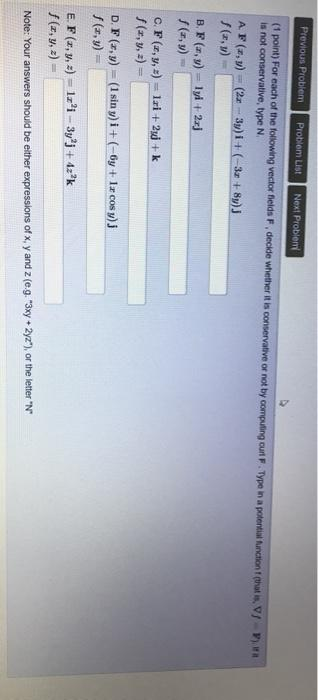### Create an Account

Already have account?

### Forgot Your Password ?

Home / Questions / Previous Problem Problem List Next Problem (1 point) For each of the following vector fiel...

# Previous Problem Problem List Next Problem (1 point) For each of the following vector fields F, decide whether it is conservative or not by computing out F. Type in a potential function that is, VJ )

Previous Problem Problem List Next Problem (1 point) For each of the following vector fields F, decide whether it is conservative or not by computing out F. Type in a potential function that is, VJ ) is not conservative, type N AF (z,y) = (2x - 3)1+ (-3x+8y)J (z,y) B. F =,y) = 1 + 2x] (:,y) C. F(, y, =) = 1x1 + 2 + k f(x, y, z) = D. F(x,y) = (1 siny)i + (-6y + 1x cosy) (y) E. F(x, y, z) = 1a'i - 3yºj + 4z"k f(x, y, z) Note: Your answers should be either expressions of x,y and z (e.g. *3xy + 2yZ"), or the letter "N"Apr 13 2021 View more View Less

#### Answer (Solved)Subscribe To Get Solution